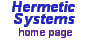Lunar Calendars
by Peter Meyer
Translations into:
German   Swedish
Ukrainian   Hungarian
Portuguese   Macedonian

# Concerning accuracy in lunar calendars

For a classification of calendars see Types of Calendars.

The synodic month (a.k.a. the mean lunar month) is the mean (that is, average) interval in days between exact conjunctions of the Moon and the Sun (as observed from the Earth). The current value of the synodic month (rounded to five decimal places) is 29.53059 days.

To describe a lunar calendar as "accurate" means that the calendar months stay in sync fairly closely with the phases of the Moon over a long period of time. If we simply used a year of thirteen calendar months alternating in lengths of 30, 29, 30, ..., 30 days then a calendar year, consisting of 384 days, would differ from thirteen mean lunar months by an average of 0.1023 days, since 13 x 29.53059 days = 383.8977 days. Thus after ten of these 13-month years the calendar would be off by about a day, and in less than half a century would be completely out of sync with the lunar cycle.

Thus some correction to the basic scheme of alternating 29- and 30-day months is needed in order that the new moon (or the full moon) should always occur on (or at least close to) the first day of the calendar month, or in another variation, that the full moon should always occur on (or at least close to) a date in the middle of the month (such as the 15th). Several attempts have been made to devise such a calendar, including the Meyer-Palmen Solilunar Calendar.

Since the length of the synodic month is changing, a problem which lunar calendar makers must face is in deciding how the synodic month will change over, say, the next 1000 years. This problem is exacerbated by the fact that the length of the day (when measured in terms of TAI, or atomic, time) is also changing (although very slowly). It is thus necessary to distinguish between two sense of the term "synodic month" (the average time, over some time period, between successive conjunctions of the Sun and the Moon when viewed from the Earth): the "observed synodic month" and the "TAI synodic month".

The TAI synodic month is given by the formula (provided by Doggett, perhaps obtained from Chapront's theory):

S = 29.5305888531 + 0.00000021621 * T - 3.64E-10 * T^2

where S is given in units of "fixed length" days of 86,400 seconds of TAI time and

T = (JD - 2,451,545) / 36,525

where JD is the Julian day number (JD 2,451,545 = 2000-01-01 CE).

For 2000-01-01 CE T = 0 so S = 29.53058885 days, and for 3000-01-01 CE T = ( 2,816,788 - 2,451,545) / 36,525 = 9.99980835, so S = 29.53059098 days. Thus according to this formula the length of the TAI synodic month is slowly increasing (and will continue to do so for thousands of years).

The observed synodic month (at some epoch) is the mean time (TAI) between successive conjunctions of the Moon and the Sun (at that epoch) divided by the number of real days which occurs in this time. Felix Verbelen (in a private communication) and Joe Kress (on the CALNDR-L mailing list) have stated how this may be calculated for 3000 CE, as follows:

The length of the day (the TAI time of the rotation of the Earth) increases by about 2 milliseconds per century (according to Stephenson's theory). If we assume lower and upper limits of 1.5 - 2.5 ms/century then the day will be longer in 3000 CE by between 0.015 and 0.025 seconds, so in 3000 CE the length of the day will thus be in the range 86,400.015 to 86,400.025 seconds (TAI).

So, for an observer on Earth, the synodic month in 3000 CE will be in the range 29.53059098 * 86400 / 86400.015 = 29.53058585 days to 29.53059098 * 86400 / 86400.025 = 29.53058244 days.

Thus the observed synodic month, in contrast to the TAI synodic month, is decreasing, and may be expected to reach about 29.53058585 real days by the year 3000 CE, and perhaps 29.53058244 real days.

Since calendars are designed to keep track of observed days and months, a lunar calendar should have an average calendar month which accords with the value of the observed synodic month, not that of the TAI synodic month. Thus a rule-based lunar calendar should have an average calendar month of, at most, the 2000 CE value of 29.53058885 days.